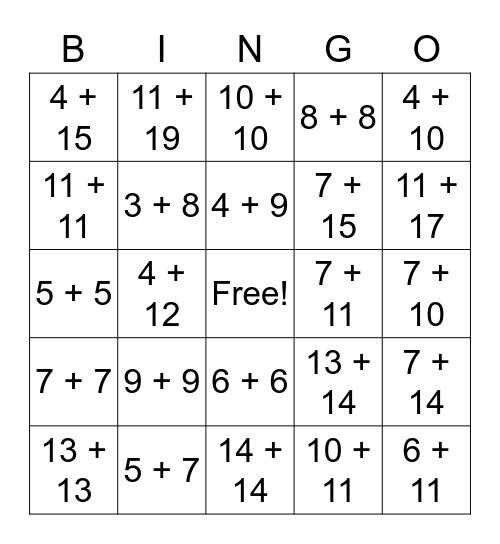This bingo card has a free space and 40 words: 4 + 6, 5 + 5, 5 + 6, 3 + 8, 5 + 7, 6 + 6, 4 + 9, 6 + 7, 4 + 10, 7 + 7, 4 + 12, 8 + 8, 6 + 11, 7 + 10, 7 + 11, 9 + 9, 9 + 10, 4 + 15, 5 + 15, 10 + 10, 7 + 14, 10 + 11, 7 + 15, 11 + 11, 6 + 17, 11 + 12, 11 + 13, 12 + 12, 10 + 15, 12 + 13, 10 + 16, 13 + 13, 19 + 8, 13 + 14, 11 + 17, 14 + 14, 10 + 19, 14 + 15, 11 + 19 and 15 + 15.

## Play Online